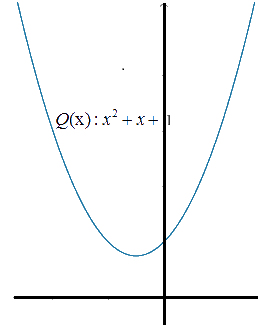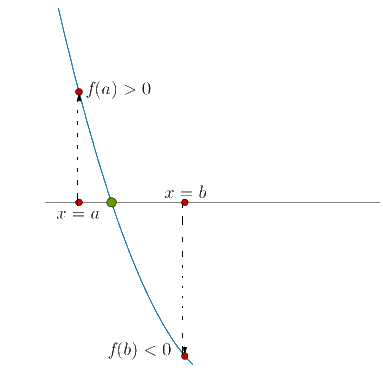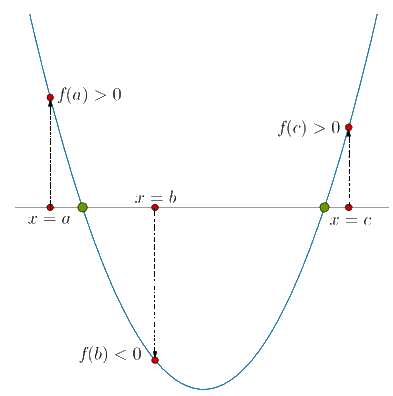Solved Example 1: Solve the inequality .

Solution: The expression $$2{x^2} + 5x + 2$$ can be factorized $$\left( {2x + 1} \right)\left( {x + 2} \right)$$, so that its zeroes are $$x = - \frac{1}{2}$$ and $$x = - 2$$. Thus, $$2{x^2} + 5x + 2 > 0$$ when

$x < - 2\,\,\,{\rm{or}}\,\,\,x > - \frac{1}{2}$

Solved Example 2: Solve the inequality $$6{x^2} - 5x + 1 < 0$$.

Solution: We have:

$\begin{array}{l}\left( {3x - 1} \right)\left( {2x - 1} \right) < 0\\ \Rightarrow \,\,\,\frac{1}{3} < x < \frac{1}{2}\end{array}$

Solved Example 3: Solve the inequality $$- {x^2} - 4x - 3 > 0$$.

Solution: We have:

$\begin{array}{l} - \left( {{x^2} + 4x + 3} \right) > 0\\ \Rightarrow \,\,\,{x^2} + 4x + 3 < 0\\ \Rightarrow \,\,\,\left( {x + 1} \right)\left( {x + 3} \right) < 0\\ \Rightarrow \,\,\, - 3 < x < - 1\end{array}$

What if the quadratic expression in an inequality has no real zeroes? Suppose that you have to find the values of x which satisfy $${x^2} + x + 1 > 0$$. The expression $${x^2} + x + 1$$ has no real zeroes. If you plot its graph, you will obtain a parabola which lies entirely above the horizontal axis:It is easy to see that $${x^2} + x + 1 > 0$$ for all values of x. Thus, the solution set of $${x^2} + x + 1 > 0$$ is the set of all real numbers.

On the other hand, the solution set of $${x^2} + x + 1 < 0$$ will be empty, because the value of $${x^2} + x + 1$$ is never negative.

Solved Example 4: Solve the inequality $${x^2} + 2x + 3 < 0$$.

Solution: We note that the discriminant for the quadratic is $$D = {2^2} - 4 \times 3 = - 8 < 0$$, so the graph of the quadratic expression $${x^2} + 2x + 3$$ never intersects the horizontal axis – in fact, it always lies above the x-axis. Thus, $${x^2} + 2x + 3 < 0$$ is satisfied for no real value of x.

Solved Example 5: Solve the inequality $$- 1 - 2x - 3{x^2} > 0$$.

Solution: We can rewrite the given inequality as follows:

$\begin{array}{l} - \left( {3{x^2} + 2x + 1} \right) > 0\\ \Rightarrow \,\,\,3{x^2} + 2x + 1 < 0\end{array}$

We note that the discrminant of the quadratic expression $$3{x^2} + 2x + 1$$ is $$D = {2^2} - 4 \times 3 = - 8 < 0$$, so the corresponding parabola will always lie above the horizontal axis. Thus, $$3{x^2} + 2x + 1 < 0$$ is satisfied for no real value of x.

Solved Example 6: Let $$f\left( x \right)$$ be a quadratic expressions. Suppose that the value of this expression is evaluated at three different points: $$x = a,\,\,x = b,\,\,x = c$$, where $$a < b < c$$. It is found that

$f\left( a \right) > 0,\,f\left( b \right) < 0,\,\,f\left( c \right) > 0$

What can you say about the zeroes of $$f\left( x \right)$$?

Solution: This problem can be solved without any calculations. Now that since $$f\left( a \right) > 0$$ and $$f\left( b \right) < 0$$, the parabola of the expression must lie above the x-axis for $$x = a$$ and below that x-axis for $$x = b$$. This means the parabola must cross the x-axis between $$x = a$$ and $$x = b$$ (as indicated by the heavy point):Similarly, $$f\left( b \right) < 0$$  and $$f\left( c \right) > 0$$, the parabola must cross the axis again between and :Thus, not only can we say that $$f\left( x \right)$$ has real and distinct zeroes, we can also specify the location of these zeroes: one zero is in the interval $$\left( {a,b} \right)$$ and the second zero is in the interval $$\left( {b,c} \right)$$. This graphical approach to problem solving in quadratics is extremely powerful!

grade 10 | Questions Set 2